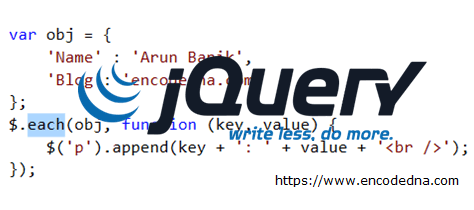﻿ jQuery - How to iterate/loop through arrays, objects and elements using .each()

# jQuery - How to iterate/loop through arrays, objects and elements using .each()

Here in this post, I am going to show you how to loop through DOM elements, arrays and objects using jQuery .each() function, something similar to forEach loop.The jQuery .each() function has many interesting properties that you can use to quickly iterate through array, objects and elememnts. At the same time using its properties to fetch and manipulate data, properties etc.

See this demo

There is a difference between the two each methods that jQuery offer.

1) \$('selector').each(), it iterates through matched elements using a selector. The selector, in this context can be any DOM element (such as <div> or <span>) or a CSS Class.

2) \$.each(), loops or iterates through jQuery objects or arrays. We’ll see both the functions in action here.

## jQuery .each() Function

Here I have four <span> elements on my web page and each has a character in it. I wish to loop through each <span>, extract the characters and append it to a <p>. It sounds like a lengthy process. However, the .each() will make it simple.

```<!DOCTYPE html>
<html>
<body>
<span>A</span>
<span>R</span>
<span>U</span>
<span>N</span>
<p></p>
</body>
<script>
// LOOP THROUGH EACH SPAN ELEMENT.
\$('span').each(function () {
\$('p').append((\$(this).text()));
});
});
</script>
</html>```

### Output

```A R U N
ARUN ```

The <span> would show four different characters. However, the <p> now shows a single word.

## Using jQuery .each() with .reverse() and .get()

We can use .each() with other jQuery functions to achieve some interesting results. I’ll use the same markup as above and read each character reverse.

```<body>

<span>A</span>
<span>R</span>
<span>U</span>
<span>N</span>
<p></p>
</body>

<script>
// Loop and show span, reverse.
\$(\$('span').get().reverse()).each(function () {
\$('p').append(\$(this).text());
});
});
</script>```

### Output

```A R U N
NURA    <-- All characters reversed.```

👉 You might also like this... How to reverse a string or a text in JavaScript using "while" loop

## jQuery .each() using Class as Selector

You can use a class name as selector to loop through elements. For example, I’ll create and add class to the <span> elements and pick values from spans that has a class property defined.

```<style>
.c1 { color: #000; }
.c2 { color: #F00; }
</style>

<body>

<p>Read values from elements that has a class defined!</p>

<span class="c1">A</span>
<span>R</span>
<span>U</span>
<span class="c1">N</span>
<p></p>
</body>
<script>
// GET VALUES OF SPANS WITH A SPECIFIC CLASS NAME.
\$('.c1').each(function () {
\$('p').append(\$(this).text());
});
});
</script>```

### Output

```A R U N
AN```

I have assigned a CSS class to the first and last <span> in the above example. Therefore, using the class as selector (.c1), it picks only the first and last span values and does the necessary manipulations on the two only.

## jQuery .each() Function with Parameters

You can pass parameters to the .each() function.

```// .each() HAS PARAMETERS.
\$('span').each(function (index, element) {
console.log(element.className + ' ' + element.tagName + ' ' + element.innerHTML);
});```

The index has an integer value that returns the index value of the elements, starting with the value 0. The parameter element (sometimes referred as just e) returns the elements properties. In the above example, it displays the class name that is attached to the <span>, the type of element (the <span>) and content in each element.

## Using jQuery .each() with Arrays

Now, this example is slightly different from the examples above. I previously used a selector with .each() function that would loop through each matching (specified) element on the page. However, now I’ll use \$.each() function to iterate through an array and retrieve the values in it.

```<script>
var arr = ['I', 'live', 'in', 'India'];
\$.each(arr, function (index, value) {
\$('p').css('fontSize', '25px');
\$('p').append(value + ' ');
});
});
</script>```

The array in the above example has four static values. The \$.each() iterates over each item in the array and appends the values to the <p>.

### Output

`I Live in India`

The index sometimes plays an important role in locating a value in the array or an object. You can filter values to limit the result, based on the indexes. For example,

```\$.each(arr, function (index, value) {
if (index == 3) {
\$('p').css('fontSize', '25px');
\$('p').append('Index: ' + index + ' has Value: ' + value);
}
});```

The output of this code will be India as I have set a condition using the index, which returns a value in the array whose index is 3.

## Using jQuery .each() with Object

The \$.each() function can work with objects too. Unlike arrays, if you are using an object as collection, the .each() function would return a key-value pair of the object.

```<script>
var obj = {
'Name' : 'Arun Banik',
'Blog': 'encodedna.com'
};
\$.each(obj, function (key, value) {
\$('p').append(key + ': ' + value + '<br />');
});
});
</script>```
Conclusion

There’s so much you can do with .each(). Use it in your app to understand the usefulness of this function. We have seen two variants of jQuery .each(), that is, \$.each() and \$(‘selector’).each() and both play an important role in an application. Not to mention, it reduces the length of your code drastically.

See this demo